### 3.3.2.1 Continuity and Balance Equations

The balance equations (2.184) to (2.186) are discretized in the same manner as POISSON's equation. Integrating over the control volumeand applying the theorem of GAUSS yields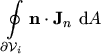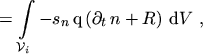(3.23)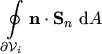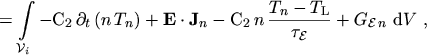(3.24)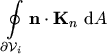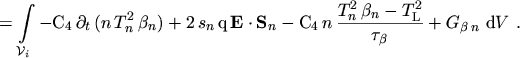(3.25)

The terms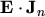and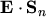are again discretized by the box integration method. Writing the electric field as the negative gradient of the electric potential and using the product rule the first term becomes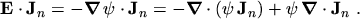(3.26)

Integration over the control volume, applying the theorem of GAUSS, and approximating the integrals by sums yields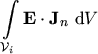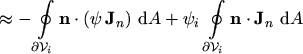(3.27)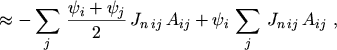(3.28)

where a linear variation of the potential between two grid points has been assumed. By combining the sums the discrete representation ofis obtained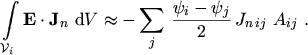(3.29)

By analogy, the discretization oflooks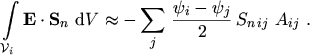(3.30)

Using eqns. (3.29) and (3.30) the discretization of the continuity equations can be concluded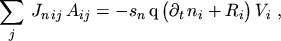(3.31)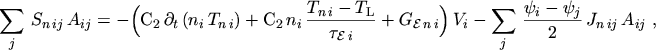(3.32)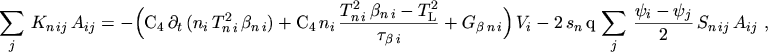(3.33)

where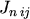,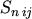,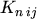are the projections of the fluxes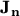,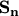,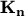onto the grid edge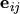.

M. Gritsch: Numerical Modeling of Silicon-on-Insulator MOSFETs PDF